## 2.3.1 Delaunay Properties

Delaunay's criterion  defines a property essential for grids used in device simulation. It is based on the definition of Voronoi boxes [95,96]. It is to note, that Delaunay's criterion and the definition of Voronoi boxes are dual, which can be seen in Fig. 2.8. The following fundamental definitions are based on definitions found in [83,88].

Definition 2..1 (Voronoi)   Let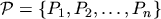be a finite set of points in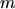-dimensional space with their location vectors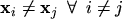. All points belong to a grid which represents the-dimensional region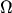. Each point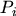is assigned a box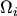defined by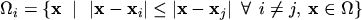called Voronoi box with its volume. The union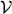of all Voronoi boxes is called Voronoi-diagram.

Fig. 2.8(a) shows the Voronoi boxwith its Volumefor a pointof a two-dimensional grid. As the two-dimensional simulations assume a constant device width in the third dimension, the actual box volume is determined by multiplication by the width. Fig. 2.8(a) can be found in many publications [45,73,74,88]. Fig. 2.8(b) shows the Voronoi boxwith its volumefor a pointof a three-dimensional grid. Both pictures clearly show, that the Voronoi boxis the set of all locations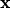which are nearer tothan to any other point of the grid.

Figure 2.8: Voronoi boxes of Delaunay grids.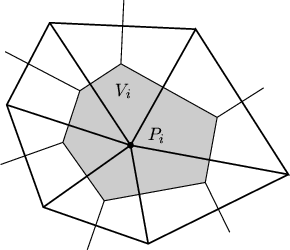(a) Two-dimensional Voronoi box.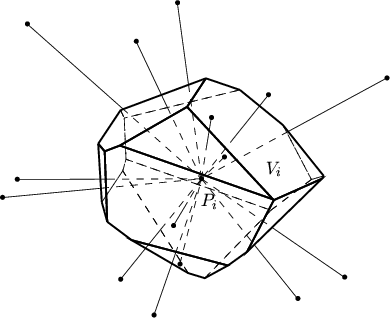(b) Three-dimensional Voronoi box.

In Fig. 2.8(a) the grid lines are drawn thick. The thin lines show the Voronoi boxes. For reasons of clearness of the picture, the line thickness has been chosen inversely in Fig. 2.8(b). The grid and the Voronoi-diagram are dual.

Definition 2..2 (Delaunay element)   Letbe a finite set of points in the-dimensional space with their location vectors. All points belong to a grid which represents the-dimensional region. Letbe an arbitrary location in. The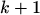linear independent points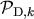with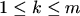form a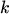-dimensional Delaunay element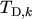if and only if there exists a locationwhich is equally close to all pointsand closer to all pointsthan to any other point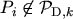. The locationthen is the center of a-dimensional sphere which passes through all points ofwhich is the-dimensional circumsphere of the Delaunay element.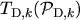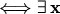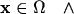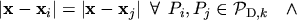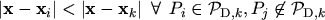A Delaunay triangle consists of Delaunay edges, a Delaunay tetrahedron consists of Delaunay triangles and Delaunay edges. Fig. 2.9(a) shows a single Delaunay triangle and its two-dimensional Voronoi boxes. The center of the circumcircle is found by the intersection of the bisecting lines of the edges. Fig. 2.9(b) shows a single Delaunay tetrahedron and its Voronoi boxes. The center of the circumsphere is the intersection of all bisecting planes of the edges.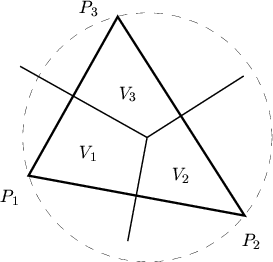(a)  Voronoi diagram of a triangle.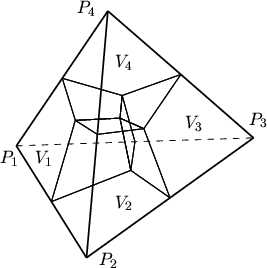(b)  Voronoi diagram of a tetrahedron.

Voronoi boxes of points at a surface propagate in the other region. For the discretization (see Section 2.4) it is necessary to truncate the box at the surface. Therefore, the surface ofis the border of the boxof the pointon the surface (see Fig. 2.10).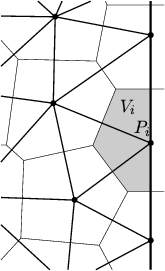Fig. 2.11 shows two possible triangulations for four given points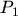,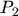,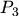, and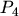. The triangulation shown in Fig. 2.11(a) is a valid Delaunay triangulation. The dual Voronoi boxes are disjunct and can be clearly assigned to their corresponding grid points.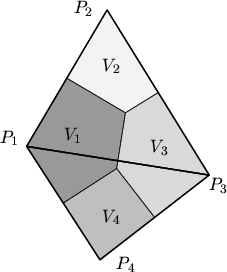(a)  Delaunay triangulation.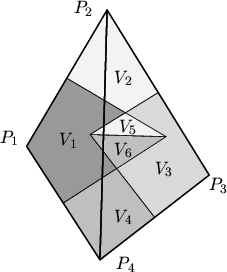(b)  Non-Delaunay triangulation.

The triangulation shown in Fig. 2.11(b) is a non-Delaunay triangulation since each circumcircle contains a point of the other triangle. The boxes are derived from the duality principle and are no Voronoi boxes. The volumes of the dual boxes are listed in Table 2.1. Because Delaunay's criterion is violated the volumes calculated are wrong which introduces an error in the discretization. In other words Voronoi boxes are desired. To calculate them the duality principle is required. For that reason Delaunay's criterion Definition 2.2 is mandatory for grids used in device simulation.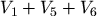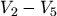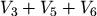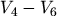Fig. 2.12(a) shows a slightly different example of a two-dimensional grid. The circumcircles shown in Fig. 2.12(b) only contain the points of the corresponding triangles. Thus, the triangulation fulfills Delaunay's criterion and the resulting boxes (Fig. 2.12(c)) are valid Voronoi boxes.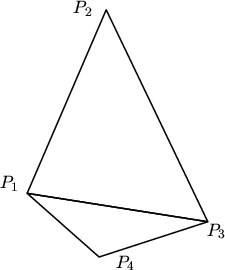(a)  Triangulation with obtuse angles.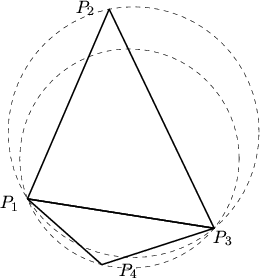(b)  Both triangles fulfill the Delaunay criterion.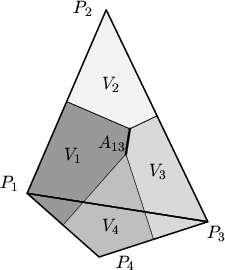(c)  Resulting Voronoi boxes.

Nevertheless, the triangulation which is caused by the obtuse angled triangle is adverse since fluxes between the pointsanare discretized using the area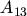which is far from the grid line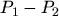. Moreover, elements like needles with no obtuse but a small angle result in a circumsphere with a large radius and cause numerical problems .

As a conclusion grids suitable for three-dimensional device simulation must consist of elements which satisfy Delaunay's criterion ( Definition 2.2). Moreover obtuse and small angled elements should be avoided.

Robert Klima 2003-02-06